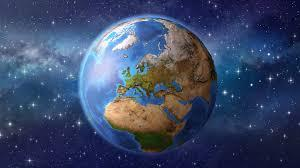# Course 16 - The Earth

9 QuestionsSettingsThe Earth A The Earth is the third planet from the Sun and it is the only planet known to have life on it. The Earth formed around 4. 5 billion years ago. It is one of four rocky planets on the inside of the Solar System. The other three are Mercury, Venus, and Mars. B The large mass of the Sun makes the Earth move around it, just as the mass of the Earth makes the Moon move around it. The Earth also turns round in space, so different parts face the Sun at different times. The Earth goes around the Sun once (one "year") for every 365¼ times it turns all the way around (one "day"). C The Moon goes around the Earth about every 27⅓ days, and reflects light from the Sun. As the Earth goes round the Sun at the same time, the cha

• 1.
The reading passage has eight paragraphs A-H. Which paragraph contains the following information? Select the correct letter, A-H. Earth's natural satellite
• A.

A

• B.

B

• C.

C

• D.

D

• E.

E

• F.

F

• G.

G

• H.

H

• 2.
Distance between Earth and Sun
• A.

A

• B.

B

• C.

C

• D.

D

• E.

E

• F.

F

• G.

G

• H.

H

• 3.
• A.

A

• B.

B

• C.

C

• D.

D

• E.

E

• F.

F

• G.

G

• H.

H

• 4.
The Solar System
• A.

A

• B.

B

• C.

C

• D.

D

• E.

E

• F.

F

• G.

G

• H.

H

• 5.
Length of most months
• A.

A

• B.

B

• C.

C

• D.

D

• E.

E

• F.

F

• G.

G

• H.

H

• 6.
Another name for Earth
• A.

A

• B.

B

• C.

C

• D.

D

• E.

E

• F.

F

• G.

G

• H.

H

• 7.
The living part of the Earth's surface
• A.

A

• B.

B

• C.

C

• D.

D

• E.

E

• F.

F

• G.

G

• H.

H

• 8.
The movements of Earth around the Sun
• A.

A

• B.

B

• C.

C

• D.

D

• E.

E

• F.

F

• G.

G

• H.

H

• 9.
Questions 9-13 Complete the sentences below. Choose NO MORE THAN THREE WORDS from the text for each answer. Apart from Earth, other rocky planets in our Solar Systems are Venus, Mars and 9[Blank]  . Moon 10[Blank]   from the Sun on Earth. There are millions of 11[Blank]   of plants and animals that inhabit Earth. Now the Solar System is travelling through 12[Blank]   . The 13[Blank]   side of the Moon is the side, which never faces Earth.Back to top